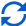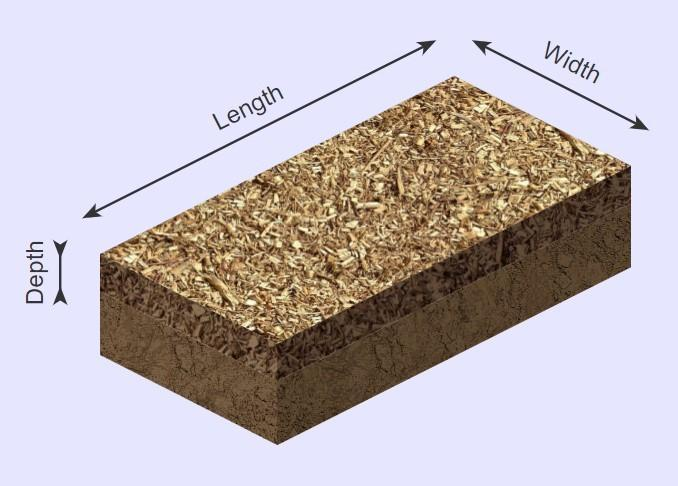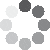Enter parameters Length & Width
Enter parameters
LENGTH, WIDTH & DEPTH
Length:
ft
• inches (in)
• feet (ft)
• yards (yd)
• centimeters (cm)
• meters (m)
Width:
ft
• inches (in)
• feet (ft)
• yards (yd)
• centimeters (cm)
• meters (m)
Depth:
in
• inches (in)
• feet (ft)
• yards (yd)
• centimeters (cm)
• meters (m)
Price per unit of volume:
yd³
• cubic yards (yd³)
• cubic feet (ft³)
• cubic inches (in³)
• cubic centimeters (cm³)
• cubic meters ()
Bag size:
ft³
• cubic inches (in³)
• cubic feet (ft³)
• cubic yards (yd³)
• cubic centimeters (cm³)
• cubic meters ()Result
Area:
0
ft²
• square feet (ft²)
• square inches (in²)
• square yards (yd²)
• square miles (mi²)
• acres (ac)
• square centimeters (cm²)
• square meters ()
Volume:
0
yd³
• cubic yards (yd³)
• cubic feet (ft³)
• cubic inches (in³)
• cubic centimeters (cm³)
• cubic meters ()
Cost:
$Bags: 0 Enter parameters AREA & DEPTH Area: ft² • square feet (ft²) • square inches (in²) • square yards (yd²) • square miles (mi²) • acres (ac) • square centimeters (cm²) • square meters () Depth: in • inches (in) • feet (ft) • yards (yd) • centimeters (cm) • meters (m) Price per unit of volume: yd³ • cubic yards (yd³) • cubic feet (ft³) • cubic inches (in³) • cubic centimeters (cm³) • cubic meters () Bag size: ft³ • cubic inches (in³) • cubic feet (ft³) • cubic yards (yd³) • cubic centimeters (cm³) • cubic meters ()Result Volume: 0 yd³ • cubic yards (yd³) • cubic feet (ft³) • cubic inches (in³) • cubic centimeters (cm³) • cubic meters () Cost:$
Bags:
0(1 votes, average: 5.00 out of 5)Loading...
Similar Calculators:
Real-time graphics. Make the calculations and see the changes.
Real-time graphics. Make the calculations and see the changes.
Embed
Similar Calculators:We think you will agree that having an idea of how many mulch chips you will need before starting a landscaping project is vital to its success.

Guessing how much mulch you will need is not easy.

You need to take into account the shape you want to fill, the density of the mulch and how deep you want the mulch coverage to be.

Estimating these values by eye can lead to over-ordering bags of mulch.

Our mulch calculator can save you both time and money.

In this article we will show you how you can use it to figure out how much mulch you need. 💪

We will also explain the Mathematical formulae it uses to calculate the total volume and cost of the landscaping project.

Contents:

## Mulch calculator formulaBased on the measurements you provide, our calculator first works out the top area (in square feet) and the volume (in cubic feet) of the garden bed using the formulae:

$$Garden\,Area = Length × Width$$

$$Garden\,Volume = Garden\,Area × Depth$$

In addition, if you know the density of the mulch you’ll be using, the calculator can work out the total weight of the required mulch:

$$Weight\,of\,Mulch = Density\,of\,Mulch × Volume$$

If you don’t know the density of the mulch, you can work it out using the formulae:

$$Density\,of\,Mulch = {Mass\,of\,Mulch \over Volume\,of\,Mulch}$$

Want to know the best part?

If you know the price per unit volume of the mulch, you can calculate the total cost using the formulae:

$$Cost = Price\,per\,Unit\,Volume × Garden\,Volume$$

If you don’t know this unit price, it’s simple enough to calculate!

$$Price\,Per\,Unit\,Volume = {Cost\,of\,Mulch \over Volume\,of\,Mulch}$$

Finally, if you know the size of a bag of mulch (for example, in cubic feet), then you can calculate how many bags you will need to purchase:

$$Number\,of\,Bags = {Garden\,Volume \over Bag\,Size}$$

Note that the number of bags should always be rounded up. Otherwise, you may end up with not enough mulch.

Confused? 😕

See below for a fully worked example where we will show you how to use the calculator.

Otherwise, simply enter your measurements into our online mulch calculator to get your next landscape project off to an efficient start!

## How to calculate mulch needed

• Let’s say that I have a rectangular garden bed which measures 20 feet in length and 8 feet wide.
• I want to ensure equal coverage of bark mulch to a depth of 4 inches.
• The cost of a 2 cubic footage bag of bark mulch is \$8.

I want to determine much mulch is required, how much it will cost and how many bags I should therefore buy.

I enter the above measurements into the calculator to work out the top area in square feet:

$$Garden\,Area = Length × Width = 20\,ft × 8\,ft = 160\,ft^2$$

and the volume of the mulch required in cubic feet to a depth of 4 inches:

$$Garden\,Volume = Garden\,Area × Depth = 160\,ft^2 × 4\,in = 53.3\,ft^3$$

To calculate the total cost, I would first need to calculate the price per unit volume:

$$Price\,Per\,Unit\,Volume = {Cost\,of\,Mulch \over Volume\,of\,Mulch} = {8 \over 2\,ft^3} = 4\,/ft^3$$

Then, the mulch calculator would work out the total cost of the mulch:

$$Cost = Price\,per\,Unit\,Volume × Garden\,Volume = 4\,/ft^3 × 53.3\,ft^3 = 213.33$$

Finally, as bags of mulch are sold in 2 cubic feet bags,

$$Number\,of\,Bags = {Garden\,Volume \over Bag\,Size} = {53.3\,ft^3 \over 2\,ft^3} = 26.6 \rightarrow 27\,bags$$

You might be wondering:

## What if my measurements aren’t in feet or inches?

Whether you want to calculate in feet, yard, meters or inches, our mulch calculator can take care of this for you.

Simply select the desired units from the drop down options and the calculator will do the following conversions for you:

$$1\,foot = 12\,inches = 0.33\,yards = 30.48\,centimeters = 0.3048\,meters$$

It couldn’t be simpler! 🙂

Embed this calculator on your site!Add live graphics
Copied to clipboard! Preview